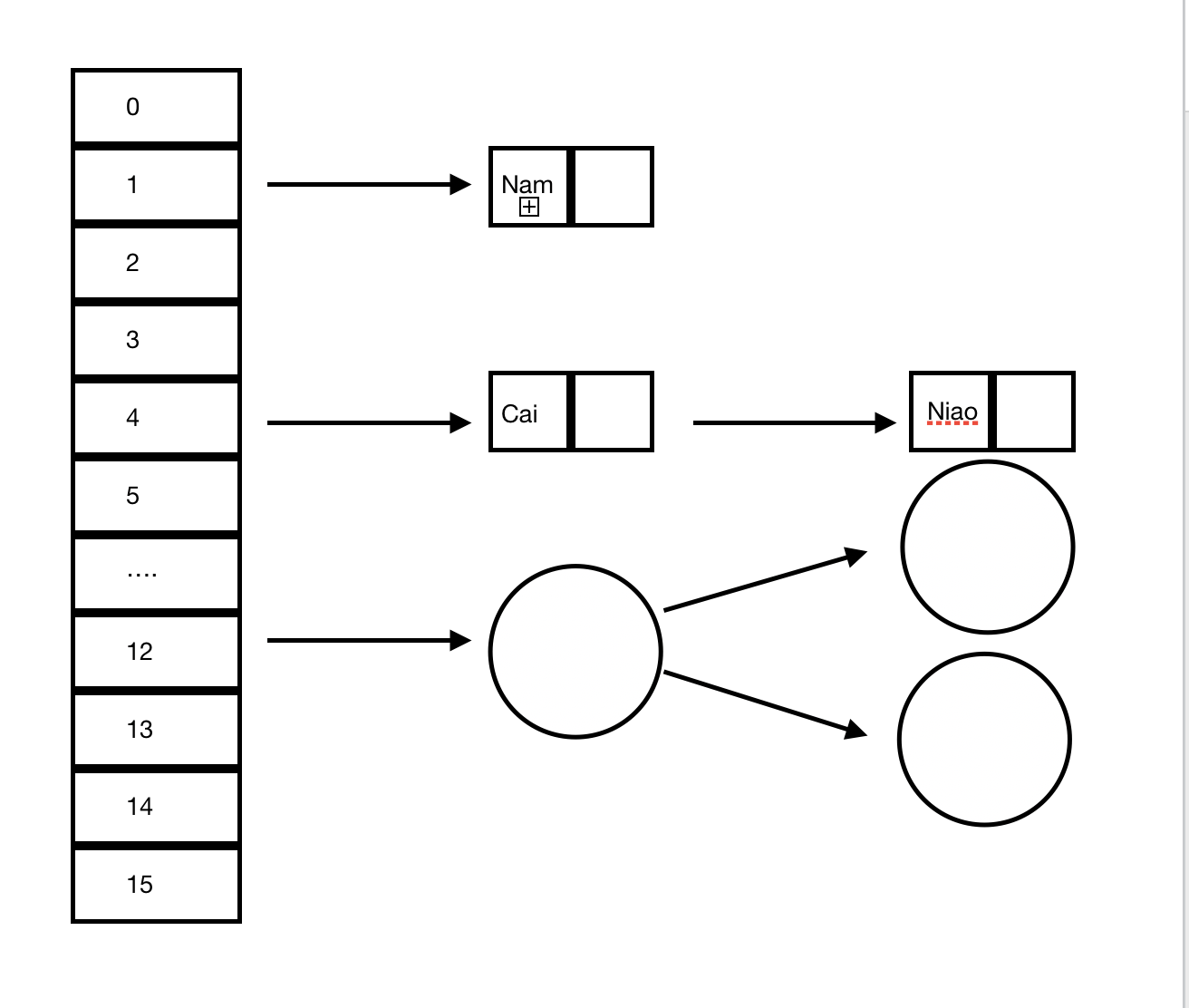# HashMap源代码分析及相关问题

P：HashMap的底层是怎么样的

Q：在JDK1.8之前 HashMap的数据结构是数组 + 链表并且链表插入方式使用了头插法，从JDK1.8开始它的数据结构就再加上了红黑树并且链表插入方式改为尾插法。

P：为什么要使用链表？

Q：因为HashMap执行put操作的时候会对键名进行hash操作，hash过后会存在hash冲突的可能，如果只使用数组不使用链表来解决哈希冲突则会将之前同hash值进行覆盖也就查询不到之前put的值。

P：后来又为什么要加上红黑树？

Q：如果hash冲突过多会造成形成的链表过长造成查询的时间过长也就是时间复杂度为O(n)，引入红黑树能够把查询的时间复杂度调整为O(lgn)。

P：什么时候又链表转换为红黑树？

Q：链表长度大于8的时候。表示默认大小，1<<4是位运算即二的四次方为16。
static final int DEFAULT_INITIAL_CAPACITY = 1 << 4;

static final int MAXIMUM_CAPACITY = 1 << 30;

static final float DEFAULT_LOAD_FACTOR = 0.75f;

static final int TREEIFY_THRESHOLD = 8;

static final int UNTREEIFY_THRESHOLD = 6;

static final int MIN_TREEIFY_CAPACITY = 64;

HashMap 添加元素过程。

//添加元素

public V put(K key, V value) {
return putVal(hash(key), key, value, false, true);
}

//对键名进行hash取值
static final int hash(Object key) {
int h;
return (key == null) ? 0 : (h = key.hashCode()) ^ (h >>> 16);
}

//添加元素
final V putVal(int hash, K key, V value, boolean onlyIfAbsent,
boolean evict) {
Node<K,V>[] tab; Node<K,V> p; int n, i;
//容量初始化，如果table为空则调用resize
if ((tab = table) == null || (n = tab.length) == 0)
n = (tab = resize()).length;
//确定元素放入哪个槽位，如果为空则新生成的结点放入槽位之中
if ((p = tab[i = (n - 1) & hash]) == null)
tab[i] = newNode(hash, key, value, null);
else {
Node<K,V> e; K k;
//比较槽位中第一个元素的hash值，key值
if (p.hash == hash &&
((k = p.key) == key || (key != null && key.equals(k))))
//如果相等则将e指向该键值对
e = p;
//如果类型为TreeNode即红黑树，则调用treeNode类中的putTreeVal方法进行插入
else if (p instanceof TreeNode)
e = ((TreeNode<K,V>)p).putTreeVal(this, tab, hash, key, value);
else {
//遍历链表
for (int binCount = 0; ; ++binCount) {
if ((e = p.next) == null) { //e到达尾节点
//新建结点，并从链表尾部插入
p.next = newNode(hash, key, value, null);
//如果到达阀值则转换为红黑树
if (binCount >= TREEIFY_THRESHOLD - 1) // -1 for 1st
treeifyBin(tab, hash);
break;
}
//如果与结点中的key相同则e指向p
if (e.hash == hash &&
((k = e.key) == key || (key != null && key.equals(k))))
break;
p = e;
}
}
//插入键值对是否存在HashMap中
if (e != null) { // existing mapping for key
V oldValue = e.value;
if (!onlyIfAbsent || oldValue == null)
e.value = value;
afterNodeAccess(e);
return oldValue;
}
}
++modCount;
//超过阀值则进行resize扩容
if (++size > threshold)
resize();
afterNodeInsertion(evict);
return null;
}

知道插入是怎么样的，那我们就来看看取值是怎么样的一个流程。

//查找结点

public V get(Object key) {
Node<K,V> e;
return (e = getNode(hash(key), key)) == null ? null : e.value;
}

//对键名进行hash取值
static final int hash(Object key) {
int h;
return (key == null) ? 0 : (h = key.hashCode()) ^ (h >>> 16);
}

//通过键名与键名hash的取值进行查找
final Node<K,V> getNode(int hash, Object key) {
Node<K,V>[] tab;
Node<K,V> first, e;
int n; K k;
//定位键值所在的位置
if ((tab = table) != null && (n = tab.length) > 0 &&
(first = tab[(n - 1) & hash]) != null) {
//如果第一结点就找到元素则返回
if (first.hash == hash && // always check first node
((k = first.key) == key || (key != null && key.equals(k))))
return first;
//如果第一结点找不到，证明多个结点
if ((e = first.next) != null) {
//如果是红黑树则调用TreeNode类的getTreeNode方法查找对应的结点。
if (first instanceof TreeNode)
return ((TreeNode<K,V>)first).getTreeNode(hash, key);
//如果不是红黑树则是链表结构，则从链表中遍历查找。
do {
if (e.hash == hash &&
((k = e.key) == key || (key != null && key.equals(k))))
return e;
} while ((e = e.next) != null);
}
}
return null;
}

如果getNode方法只使用hash来查找能否准确找到要取出的那个值？10-081万+

#### hashmap转红黑树的两个条件11-09170

#### 一篇文章彻底读懂HashMap之HashMap源码解析（上）

03-051730

#### HashMap的put源码解析

06-0158

#### HashMap的put方法源码解析及其中两种会触发扩容的场景(足够详尽，有问题欢迎指正~)

08-19327

#### JDK1.8中关于hashMap中的红黑树的学习

06-07534

#### JDK1.8 HashMap源码解析（不分析红黑树部分）

03-2852

#### 【JDK1.8源码阅读】关于HashMap你应该知道的

06-20166

#### 一文搞定HashMap的实现原理和面试

04-101万+

#### HashMap在jdk1.8为何引入了红黑树?©️2020 CSDN 皮肤主题: 数字20 设计师: CSDN官方博客点击重新获取扫码支付1.余额是钱包充值的虚拟货币，按照1:1的比例进行支付金额的抵扣。
2.余额无法直接购买下载，可以购买VIP、C币套餐、付费专栏及课程。余额充值